### Home > PC > Chapter 9 > Lesson 9.1.3 > Problem9-45

9-45.
1. Given f(x) = 10x2 and g(x) = 15 − 3x, find the following: Homework Help ✎

1. f(3 + h) − 90

2. f(x + h) − 10x2

3. g(20 + h)

4. f(g(x))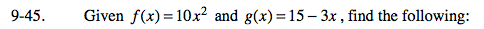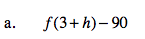Substitute
(3 + h) for every 'x' in the 'f' function. Simplify.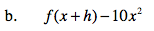20xh + 10h2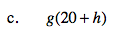Substitute (20+h) for every 'x' in the 'g' function. Simplify.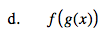Substitute
(15 − 3x) for every 'x' in the 'f' function. Simplify.

2250 − 900x + 90x2